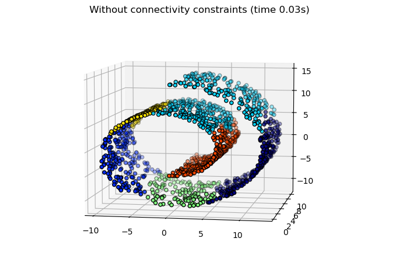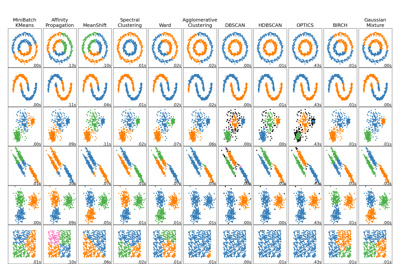# sklearn.neighbors.kneighbors_graph¶

sklearn.neighbors.kneighbors_graph(X, n_neighbors, *, mode='connectivity', metric='minkowski', p=2, metric_params=None, include_self=False, n_jobs=None)[source]

Computes the (weighted) graph of k-Neighbors for points in X

Read more in the User Guide.

Parameters
Xarray-like of shape (n_samples, n_features) or BallTree

Sample data, in the form of a numpy array or a precomputed BallTree.

n_neighborsint

Number of neighbors for each sample.

mode{‘connectivity’, ‘distance’}, default=’connectivity’

Type of returned matrix: ‘connectivity’ will return the connectivity matrix with ones and zeros, and ‘distance’ will return the distances between neighbors according to the given metric.

metricstr, default=’minkowski’

The distance metric used to calculate the k-Neighbors for each sample point. The DistanceMetric class gives a list of available metrics. The default distance is ‘euclidean’ (‘minkowski’ metric with the p param equal to 2.)

pint, default=2

Power parameter for the Minkowski metric. When p = 1, this is equivalent to using manhattan_distance (l1), and euclidean_distance (l2) for p = 2. For arbitrary p, minkowski_distance (l_p) is used.

metric_paramsdict, default=None

additional keyword arguments for the metric function.

include_selfbool or ‘auto’, default=False

Whether or not to mark each sample as the first nearest neighbor to itself. If ‘auto’, then True is used for mode=’connectivity’ and False for mode=’distance’.

n_jobsint, default=None

The number of parallel jobs to run for neighbors search. None means 1 unless in a joblib.parallel_backend context. -1 means using all processors. See Glossary for more details.

Returns
Asparse matrix of shape (n_samples, n_samples)

Graph where A[i, j] is assigned the weight of edge that connects i to j. The matrix is of CSR format.

Examples

>>> X = [, , ]
>>> from sklearn.neighbors import kneighbors_graph
>>> A = kneighbors_graph(X, 2, mode='connectivity', include_self=True)
>>> A.toarray()
array([[1., 0., 1.],
[0., 1., 1.],
[1., 0., 1.]])


## Examples using sklearn.neighbors.kneighbors_graph¶